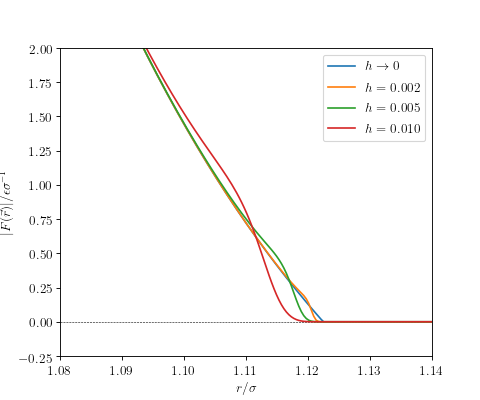# Potential truncations¶

## sharp¶

Create sharp truncation by setting the potential to zero for particle distances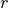larger than the cutoff distance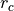, i.e., by multiplying with a step function: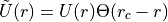Both potential and force are discontinuous at the cutoff.

Example:

potential = potential:truncate({"sharp", cutoff = 4})


## shifted¶

Amend the sharp truncation by an energy shift such that the potential is continuous at the cutoff distance: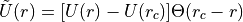The force is not affected by this and remains discontinuous at the cutoff.

Example:

potential = potential:truncate({"shifted", cutoff = 2.5})


## force_shifted¶

Amend the energy-shifted potential by a linear term, effectively shifting the force to zero at the cutoff distance: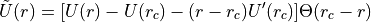Both energy and force are continuous at the cutoff. Note that this modification globally tilts the potential with possible physical implications for, e.g., phase diagrams.

Example:

potential = potential:truncate({"force_shifted", cutoff = 2.5})


## smooth_r4¶

Truncate the potential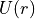such that it remains a C²-continuous function at the cutoff, i.e., the force being continuously differentiable. As a consequence, momentum and energy drift are drastically diminished even from long runs using symplectic integrators such as halmd.mdsim.integrators.verlet.

The truncation is implemented by multiplication of the energy-shifted potential with the local smoothing function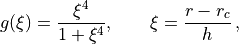whereis the cutoff distance, and the parameter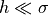, which has the dimension of a length, controls the the range of smoothing. The C²-continuous truncated potential then readsand the C¹-continuous force is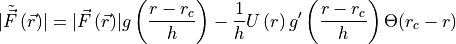with the derivative of the smoothing function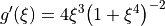.

Example:

potential = potential:truncate({"smooth_r4", cutoff = 2.5, h = 0.005})


The following figure shows unmodified and C²-smooth variants of the Weeks-Chandler-Andersen potential, the repulsive part of the Lennard-Jones potential sharply cutoff at.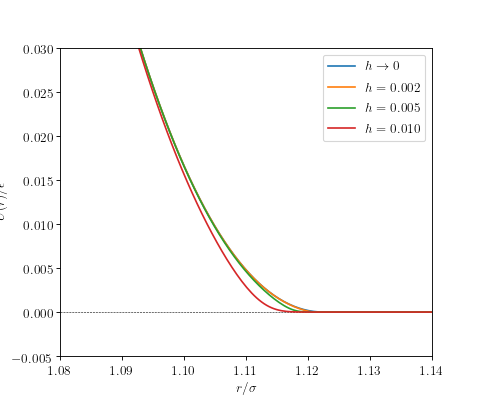The following figure shows the absolute value of the force.